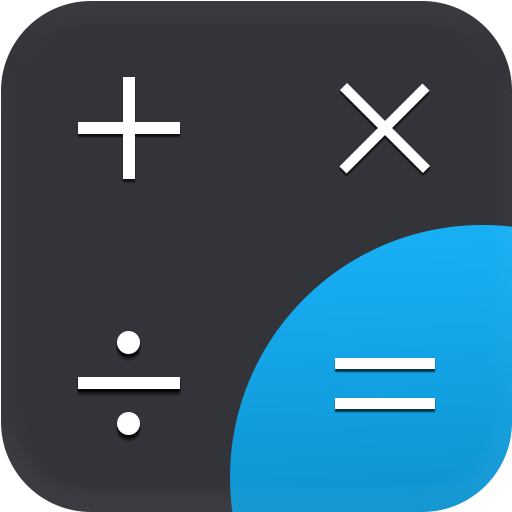### Description

Scientific Calculator solve any mathematics problem easily.

The original All-In-One Scientific Calculator for Android.

It's a FREE, complete and easy to use multi calculator & converter.

Scientific Calculator is suitable for solving easy common calculus and more complex mathematical calculations.

Designed with simplicity in mind, it helps you solve everyday problems.
From simple or complex calculations, to unit and currency conversions, percentages, proportions, areas, volumes, etc... it does it all. And it does it good!

Simply the the best free scientific calculator on the market.

Scientific Calculator has many facilities, such as:
- basic arithmetic operations including percentage, modulo, etc.
- fractions (in the expression mode any expression including nested fractions can be entered as a numerator and a denominator).
- mixed numbers.
- Pi
- periodic numbers and their conversion to fractions.
- operator priority.
- common unit conversions
- conversion between rectangular and polar coordinates
- advanced number operations such as random numbers, combinations, permutations, common greatest divisor, etc.
- trigonometric and hyperbolic functions.
- powers, roots, logarithms, etc.
- Mean, Sum, # Elements
- Standard Deviation
- Log, Ln, Inverse Log, Exponential
- nPr, cPr, x!
- Random Number Generator
- degrees, minutes and seconds conversion.
- fixed point, scientific and engineering display format.
- Three different modes: Float, Fixed and Scientific
- display exponent as SI units prefix.
- memory operations with 10 extended memories.
- clipboard operations with various clipboard formats.
- result history.
- logical operations.
- bitwise shifts and rotations.
- Reverse Polish notation.
- can perform multiple complex calculation in single step
- works offline, no internet connection required
- binary, octal, and hexadecimal (can be enabled in Settings)
- Very high internal precision. For calculations like: cubeRoot(17)*cubeRoot(17)*cubeRoot(17) = 17
-Switch between the scientific calculator layout and the simple layout by rotating your device.

Scientific Calculator used to calculate every equations in seconds.

Primary: Applications

Secondary: Tools

### Total Ratings

11
 5 7 4 1 3 0 2 0 1 3

### Screenshots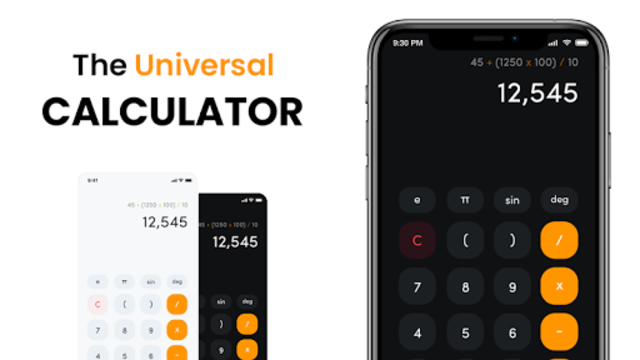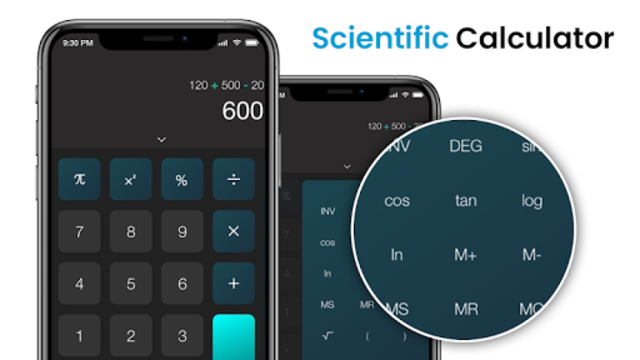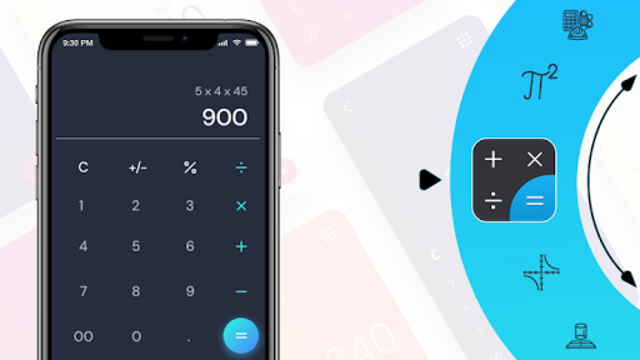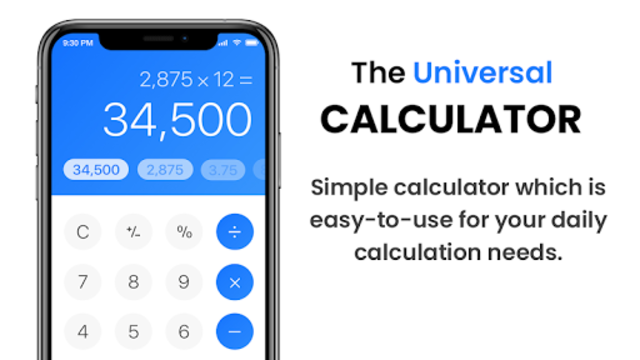### Version History

Launched Apr 27, 2020 (9 months ago).

Releasing new versions every about 2 months, on average.

 Oct 062020 (Current)Version 1.1.3Added more mathematical and statistical formulas. Oct 042020 Version 1.1.2Added more mathematical and statistical formulas. Oct 012020 Version 1.1.1Ads ratio decrease. Apr 262020 Version 1.0.9 Click to see more